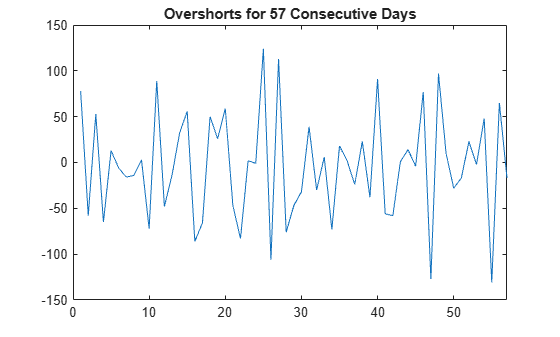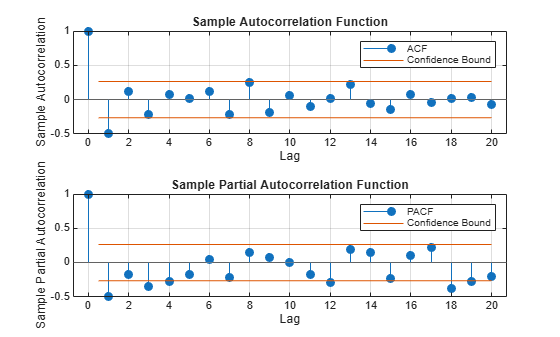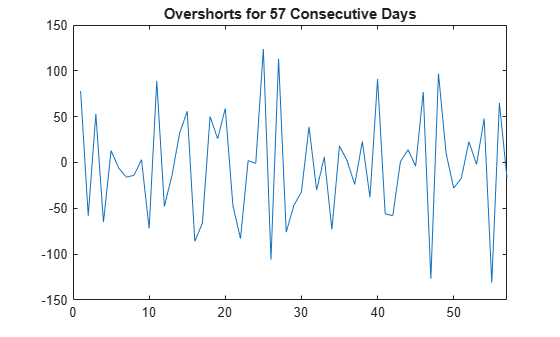Documentation

## Detect Autocorrelation

### Compute Sample ACF and PACF

This example shows how to compute the sample autocorrelation function (ACF) and partial autocorrelation function (PACF) to qualitatively assess autocorrelation.

The time series is 57 consecutive days of overshorts from a gasoline tank in Colorado.

Load the time series of overshorts.

```load(fullfile(matlabroot,'examples','econ','Data_Overshort.mat')) Y = Data; N = length(Y); figure plot(Y) xlim([0,N]) title('Overshorts for 57 Consecutive Days')```The series appears to be stationary.

Step 2. Plot the sample ACF and PACF.

Plot the sample autocorrelation function (ACF) and partial autocorrelation function (PACF).

```figure subplot(2,1,1) autocorr(Y) subplot(2,1,2) parcorr(Y)```The sample ACF and PACF exhibit significant autocorrelation. The sample ACF has significant autocorrelation at lag 1. The sample PACF has significant autocorrelation at lags 1, 3, and 4.

The distinct cutoff of the ACF combined with the more gradual decay of the PACF suggests an MA(1) model might be appropriate for this data.

Step 3. Store the sample ACF and PACF values.

Store the sample ACF and PACF values up to lag 15.

```acf = autocorr(Y,'NumLags',15); pacf = parcorr(Y,'NumLags',15); [length(acf) length(pacf)]```
```ans = 1×2 16 16 ```

The outputs `acf` and `pacf` are vectors storing the sample autocorrelation and partial autocorrelation at lags 0, 1,...,15 (a total of 16 lags).

### Conduct the Ljung-Box Q-Test

This example shows how to conduct the Ljung-Box Q-test for autocorrelation.

The time series is 57 consecutive days of overshorts from a gasoline tank in Colorado.

Load the time series of overshorts.

```load(fullfile(matlabroot,'examples','econ','Data_Overshort.mat')) Y = Data; N = length(Y); figure plot(Y) xlim([0,N]) title('Overshorts for 57 Consecutive Days')```The data appears to fluctuate around a constant mean, so no data transformations are needed before conducting the Ljung-Box Q-test.

Step 2. Conduct the Ljung-Box Q-test.

Conduct the Ljung-Box Q-test for autocorrelation at lags 5, 10, and 15.

`[h,p,Qstat,crit] = lbqtest(Y,'Lags',[5,10,15])`
```h = 1x3 logical array 1 1 1 ```
```p = 1×3 0.0016 0.0007 0.0013 ```
```Qstat = 1×3 19.3604 30.5986 36.9639 ```
```crit = 1×3 11.0705 18.3070 24.9958 ```

All outputs are vectors with three elements, corresponding to tests at each of the three lags. The first element of each output corresponds to the test at lag 5, the second element corresponds to the test at lag 10, and the third element corresponds to the test at lag 15.

The test decisions are stored in the vector `h`. The value `h = 1` means reject the null hypothesis. Vector `p` contains the p-values for the three tests. At the $\alpha =0.05$ significance level, the null hypothesis of no autocorrelation is rejected at all three lags. The conclusion is that there is significant autocorrelation in the series.

The test statistics and ${\chi }^{2}$ critical values are given in outputs `Qstat` and `crit`, respectively.

 Brockwell, P. J. and R. A. Davis. Introduction to Time Series and Forecasting. 2nd ed. New York, NY: Springer, 2002.